﻿

### On the ranks of homogeneous polynomials of degree at least 9 and border rank 5

#### Abstract

Let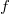$f$ be a degree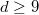$d \ge 9$ homogenous polynomial with border rank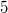$5$. We prove that it has rank at most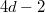$4d-2$ and give better results when$f$ essentially depends on at most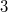$3$ variables or there are other conditions on the scheme evincing the cactus and border rank of$f$. We always assume that$f$ essentially depends on at most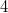$4$ variables, because the other case was done by myself in Acta Math. Vietnam. 42 (2017), 509-531.

DOI Code: 10.1285/i15900932v38n2p55

Keywords: symmetric tensor rank; border rank; cactus rank

Full Text: PDF